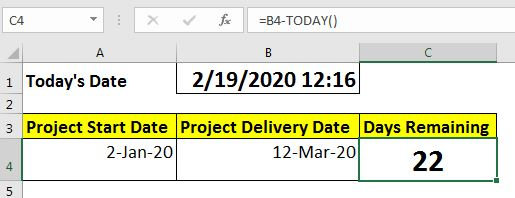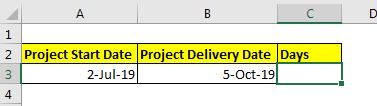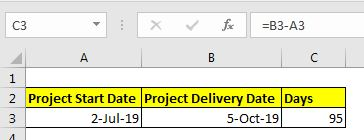# How to Countdown Remaining Days in ExcelWhile working with date-based data, you often get the need to countdown the remaining days from one date to another date. In this article, we will learn how to count the days between two dates.

Generic Formula:

 =Later Date - Earlier Date

Here,

Later Date: This is an excel date that comes later than the Earlier Date.

Earlier Date: Earlier Date is the date before the Later Date. You can replace it with TODAY() function to make days countdown formula. We will see how soon in this article.

So, let's take an example to make everything clear.

## Example: Count the number of days between the project start date and expected delivery date:

So the task is this. I have a project start date and expected delivery date. I just need to calculate the days between the start date and delivery date:Here later date is Project Delivery Date and Earlier Date is Project Start date. So, if we implement the above generic formula then we will get this formula:

 =B3- A3And this will return 95. Hence 95 days are between the project start date and end date. If you want to calculate the remaining working days then read this.

What if you want to get the remaining days before the project delivery date?

In the above solution, we calculated the days between the start date and delivery date. But if you want to create a function to countdown the remaining days before the project delivery date, then it won't work. The solution is the TODAY function. Let's see how.

So here I have a project start date and delivery date. I want a countdown cell that tells me the remaining days till the delivery date. The formula will be:

 =B4-TODAY()How does it work?

The method is simple. In Excel, dates are nothing but serial numbers. The serial starts from 1 Jan 1900. Each day counts one. So, if you subtract an earlier date (small number)  from Later Date (larger number), you will get the number of days between the two dates. You can read about Excel dates in detail here.

In the first example, we simply subtract the past date from the future date. This returns the date days between the two dates.

In the day countdown example, I subtract TODAY() from the future date. The TODAY function returns today's date which is obviously less than the future date (if it is not then you are in trouble). This returns the days remains till the delivery date.

Related Articles

How to use the EDATE function in Excel |EDATE function returns the date after adding or subtracting months on a given date. The EDATE function takes the date as an argument and edits n months to the date.

Calculate age from date of birth | To calculate age we use the TODAY function of excel. We subtract the date of birth from today's date.

How to Group Data By Age Range in Excel | To group data by age in the pivot, follow the simple steps explained here. All the data will be arranged in a mannered way.

Popular Articles:

50 Excel Shortcuts to Increase Your Productivity | Get faster at your task. These 50 shortcuts will make you work even faster on Excel.

How to use Excel VLOOKUP Function| This is one of the most used and popular functions of excel that is used to lookup value from different ranges and sheets.

How to use the Excel COUNTIF Function| Count values with conditions using this amazing function. You don't need to filter your data to count specific value. Countif function is essential to prepare your dashboard.

How to Use SUMIF Function in Excel | This is another dashboard essential function. This helps you sum up values on specific conditions.

Terms and Conditions of use

The applications/code on this site are distributed as is and without warranties or liability. In no event shall the owner of the copyrights, or the authors of the applications/code be liable for any loss of profit, any problems or any damage resulting from the use or evaluation of the applications/code.